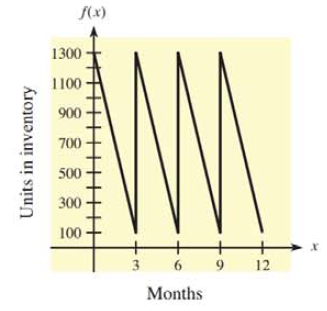Chapter 13.3, Problem 38E### Mathematical Applications for the ...

11th Edition
Ronald J. Harshbarger + 1 other
ISBN: 9781305108042

#### Solutions

Chapter
Section### Mathematical Applications for the ...

11th Edition
Ronald J. Harshbarger + 1 other
ISBN: 9781305108042
Textbook Problem

# Inventory management The figure shows how an inventory of a product is depleted each quarter of a given year. What is the average inventory per month for the first 3 months for this product? [Assume that the graph is a line joining ( 0, 1300 )  and  ( 3, 100 ) .]To determine

To calculate: The average inventory per month for the first 3 months where how an inventory of a product is depleted each quarter of a given year is shown by the figure.Explanation

Given information:

The provided figure is:

Formula used:

Equation of line:

The equation of a line passing through the points (x1,y1) and (x2,y2) is given as:

(yy1)=y2y1x2x1(xx1)

Average value

The average value of a continuous function y=f(x) over the interval [a,b] is:

Average value=1baabf(x)dx

Calculation:

Consider the figure:

The average inventory per month for the first 3 months is to be calculated.

This inventory is given by the equation which passes through (0,1300) and (3,100)

Consider the formula:

(yy1)=y2y1x2x1(xx1)

Substitute 1300 for y2, 100 for y1, 3 for x1 and 0 for x1 in above equation to get:

(y100)=130010003(x3)y100=12003(x3)y=400x+1200+

### Still sussing out bartleby?

Check out a sample textbook solution.

See a sample solution

#### The Solution to Your Study Problems

Bartleby provides explanations to thousands of textbook problems written by our experts, many with advanced degrees!

Get Started

#### Evaluate the expression sin Exercises 116. 2322

Finite Mathematics and Applied Calculus (MindTap Course List)

#### Differentiate the function. H(z)=a2z2a2+z2

Single Variable Calculus: Early Transcendentals, Volume I

#### In Exercises 5-8, find the values of the variables. [1xy3]=[z23w]

Finite Mathematics for the Managerial, Life, and Social Sciences